## 2.5 Limits at Infinity; Asymptotes of Graphs

### Learning Objectives

• Calculate the limit of a function as $x$ increases or decreases without bound.
• Using correct notation, describe an infinite limit.
• Define a vertical asymptote.
• Recognize a horizontal asymptote on the graph of a function.
• Estimate the end behavior of a function as $x$ increases or decreases without bound.
• Recognize an oblique asymptote on the graph of a function.

Infinite Limits

Evaluating the limit of a function at a point or evaluating the limit of a function from the right and left at a point helps us to characterize the behavior of a function around a given value. As we shall see, we can also describe the behavior of functions that do not have finite limits.

We now turn our attention to $h(x)=1/(x-2)^2$. From its graph we see that as the values of $x$ approach 2, the values of $h(x)=1/(x-2)^2$ become larger and larger and, in fact, become infinite. Mathematically, we say that the limit of $h(x)$ as $x$ approaches 2 is positive infinity. Symbolically, we express this idea as

$\underset{x\to 2}{\lim}h(x)=+\infty$.

More generally, we define infinite limits as follows:

### Definition

We define three types of infinite limits.

Infinite limits from the left: Let $f(x)$ be a function defined at all values in an open interval of the form $(b,a)$.

1. If the values of $f(x)$ increase without bound as the values of $x$ (where $x<a$) approach the number $a$, then we say that the limit as $x$ approaches $a$ from the left is positive infinity and we write
$\underset{x\to a^-}{\lim}f(x)=+\infty$.
2. If the values of $f(x)$ decrease without bound as the values of $x$ (where $x<a$) approach the number $a$, then we say that the limit as $x$ approaches $a$ from the left is negative infinity and we write
$\underset{x\to a^-}{\lim}f(x)=−\infty$.

Infinite limits from the right: Let $f(x)$ be a function defined at all values in an open interval of the form $(a,c)$.

1. If the values of $f(x)$ increase without bound as the values of $x$ (where $x>a$) approach the number $a$, then we say that the limit as $x$ approaches $a$ from the left is positive infinity and we write
$\underset{x\to a^+}{\lim}f(x)=+\infty$.
2. If the values of $f(x)$ decrease without bound as the values of $x$ (where $x>a$) approach the number $a$, then we say that the limit as $x$ approaches $a$ from the left is negative infinity and we write
$\underset{x\to a^+}{\lim}f(x)=−\infty$.

Two-sided infinite limit: Let $f(x)$ be defined for all $x\ne a$ in an open interval containing $a$.

1. If the values of $f(x)$ increase without bound as the values of $x$ (where $x\ne a$) approach the number $a$, then we say that the limit as $x$ approaches $a$ is positive infinity and we write
$\underset{x\to a}{\lim}f(x)=+\infty$.
2. If the values of $f(x)$ decrease without bound as the values of $x$ (where $x\ne a$) approach the number $a$, then we say that the limit as $x$ approaches $a$ is negative infinity and we write
$\underset{x\to a}{\lim}f(x)=−\infty$.

It is important to understand that when we write statements such as $\underset{x\to a}{\lim}f(x)=+\infty$ or $\underset{x\to a}{\lim}f(x)=−\infty$ we are describing the behavior of the function, as we have just defined it. We are not asserting that a limit exists. For the limit of a function $f(x)$ to exist at $a$, it must approach a real number $L$ as $x$ approaches $a$. That said, if, for example, $\underset{x\to a}{\lim}f(x)=+\infty$, we always write $\underset{x\to a}{\lim}f(x)=+\infty$ rather than $\underset{x\to a}{\lim}f(x)$ DNE.

### Recognizing an Infinite Limit

Evaluate each of the following limits, if possible. Use a table of functional values and graph $f(x)=1/x$ to confirm your conclusion.

1. $\underset{x\to 0^-}{\lim}\frac{1}{x}$
2. $\underset{x\to 0^+}{\lim}\frac{1}{x}$
3. $\underset{x\to 0}{\lim}\frac{1}{x}$

Evaluate each of the following limits, if possible. Use a table of functional values and graph $f(x)=1/x^2$ to confirm your conclusion.

1. $\underset{x\to 0^-}{\lim}\frac{1}{x^2}$
2. $\underset{x\to 0^+}{\lim}\frac{1}{x^2}$
3. $\underset{x\to 0}{\lim}\frac{1}{x^2}$

#### Hint

Follow the procedures from (Figure).

### Evaluating a Limit of the Form $K/0, \, K\ne 0$ Using the Limit Laws

Evaluate $\underset{x\to 2^-}{\lim}\frac{x-3}{x^2-2x}$.

Evaluate $\underset{x\to 1}{\lim}\frac{x+2}{(x-1)^2}$.

#### Hint

Use the methods from (Figure).

It is useful to point out that functions of the form $f(x)=1/(x-a)^n$, where $n$ is a positive integer, have infinite limits as $x$ approaches $a$ from either the left or right ((Figure)). These limits are summarized in (Figure).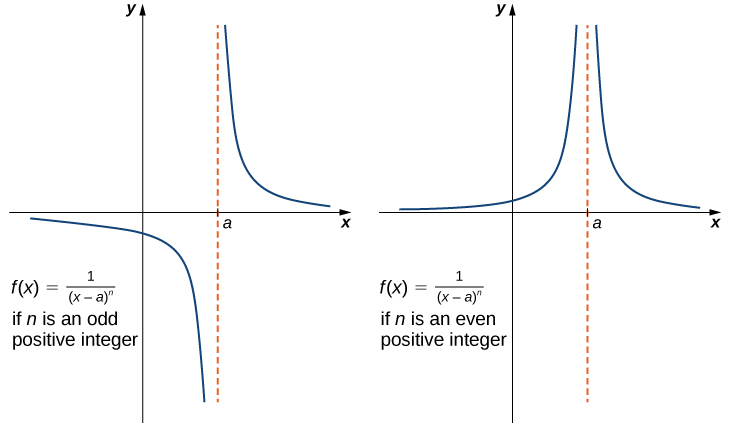Figure 9. The function $f(x)=1/(x-a)^n$ has infinite limits at $a$.

### Infinite Limits from Positive Integers

If $n$ is a positive even integer, then

$\underset{x\to a}{\lim}\frac{1}{(x-a)^n}=+\infty$.

If $n$ is a positive odd integer, then

$\underset{x\to a^+}{\lim}\frac{1}{(x-a)^n}=+\infty$

and

$\underset{x\to a^-}{\lim}\frac{1}{(x-a)^n}=−\infty$.

We should also point out that in the graphs of $f(x)=1/(x-a)^n$, points on the graph having $x$-coordinates very near to $a$ are very close to the vertical line $x=a$. That is, as $x$ approaches $a$, the points on the graph of $f(x)$ are closer to the line $x=a$. The line $x=a$ is called a vertical asymptote of the graph. We formally define a vertical asymptote as follows:

### Definition

Let $f(x)$ be a function. If any of the following conditions hold, then the line $x=a$ is a vertical asymptote of $f(x)$:

$\begin{array}{ccc}\hfill \underset{x\to a^-}{\lim}f(x)& =\hfill & +\infty \, \text{or} \, -\infty \hfill \\ \hfill \underset{x\to a^+}{\lim}f(x)& =\hfill & +\infty \, \text{or} \, −\infty \hfill \\ & \text{or}\hfill & \\ \hfill \underset{x\to a}{\lim}f(x)& =\hfill & +\infty \, \text{or} \, −\infty \hfill \end{array}$

### Finding a Vertical Asymptote

Evaluate each of the following limits using (Figure). Identify any vertical asymptotes of the function $f(x)=1/(x+3)^4$.

1. $\underset{x\to -3^-}{\lim}\frac{1}{(x+3)^4}$
2. $\underset{x\to -3^+}{\lim}\frac{1}{(x+3)^4}$
3. $\underset{x\to -3}{\lim}\frac{1}{(x+3)^4}$

Evaluate each of the following limits. Identify any vertical asymptotes of the function $f(x)=\frac{1}{(x-2)^3}$.

1. $\underset{x\to 2^-}{\lim}\frac{1}{(x-2)^3}$
2. $\underset{x\to 2^+}{\lim}\frac{1}{(x-2)^3}$
3. $\underset{x\to 2}{\lim}\frac{1}{(x-2)^3}$

#### Hint

Use (Figure).

In the next example we put our knowledge of various types of limits to use to analyze the behavior of a function at several different points.

### Behavior of a Function at Different Points

Use the graph of $f(x)$ in (Figure) to determine each of the following values:

1. $\underset{x\to 3^-}{\lim}f(x); \, \underset{x\to 3^+}{\lim}f(x); \, \underset{x\to 3}{\lim}f(x); \, f(3)$
2. $\underset{x\to \infty}{\lim}f(x)$
3. $\underset{x\to -\infty}{\lim}f(x)$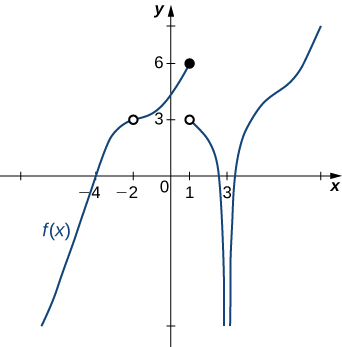Figure 10. The graph shows $f(x)$.

Evaluate $\underset{x\to 1}{\lim}f(x)$ for $f(x)$ shown here: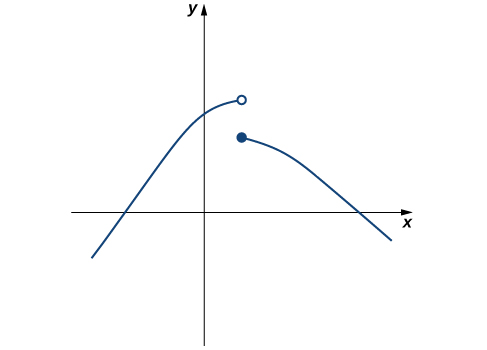#### Hint

Compare the limit from the right with the limit from the left.

## Limits at Infinity and Horizontal Asymptotes

Recall that $\underset{x \to a}{\lim}f(x)=L$ means $f(x)$ becomes arbitrarily close to $L$ as long as $x$ is sufficiently close to $a$. We can extend this idea to limits at infinity. For example, consider the function $f(x)=2+\frac{1}{x}$. As can be seen graphically in (Figure) and numerically in (Figure), as the values of $x$ get larger, the values of $f(x)$ approach 2. We say the limit as $x$ approaches $\infty$ of $f(x)$ is 2 and write $\underset{x\to \infty }{\lim}f(x)=2$. Similarly, for $x<0$, as the values $|x|$ get larger, the values of $f(x)$ approaches 2. We say the limit as $x$ approaches $−\infty$ of $f(x)$ is 2 and write $\underset{x\to a}{\lim}f(x)=2$.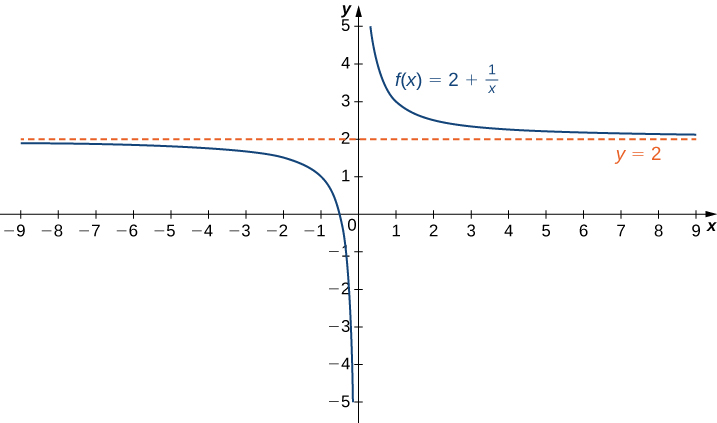Figure 1. The function approaches the asymptote $y=2$ as $x$ approaches $\pm \infty$.

 $x$ 10 100 1,000 10,000 $2+\frac{1}{x}$ 2.1 2.01 2.001 2.0001 $x$ -10 -100 -1000 -10,000 $2+\frac{1}{x}$ 1.9 1.99 1.999 1.9999

More generally, for any function $f$, we say the limit as $x \to \infty$ of $f(x)$ is $L$ if $f(x)$ becomes arbitrarily close to $L$ as long as $x$ is sufficiently large. In that case, we write $\underset{x\to a}{\lim}f(x)=L$. Similarly, we say the limit as $x\to −\infty$ of $f(x)$ is $L$ if $f(x)$ becomes arbitrarily close to $L$ as long as $x<0$ and $|x|$ is sufficiently large. In that case, we write $\underset{x\to −\infty }{\lim}f(x)=L$. We now look at the definition of a function having a limit at infinity.

### Definition

(Informal) If the values of $f(x)$ become arbitrarily close to $L$ as $x$ becomes sufficiently large, we say the function $f$ has a limit at infinity and write

$\underset{x\to \infty }{\lim}f(x)=L$.

If the values of $f(x)$ becomes arbitrarily close to $L$ for $x<0$ as $|x|$ becomes sufficiently large, we say that the function $f$ has a limit at negative infinity and write

$\underset{x\to -\infty }{\lim}f(x)=L$.

If the values $f(x)$ are getting arbitrarily close to some finite value $L$ as $x\to \infty$ or $x\to −\infty$, the graph of $f$ approaches the line $y=L$. In that case, the line $y=L$ is a horizontal asymptote of $f$ ((Figure)). For example, for the function $f(x)=\frac{1}{x}$, since $\underset{x\to \infty }{\lim}f(x)=0$, the line $y=0$ is a horizontal asymptote of $f(x)=\frac{1}{x}$.

### Definition

If $\underset{x\to \infty }{\lim}f(x)=L$ or $\underset{x \to −\infty}{\lim}f(x)=L$, we say the line $y=L$ is a horizontal asymptote of $f$.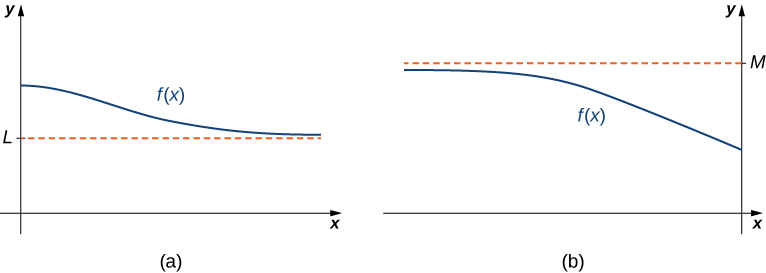Figure 2. (a) As $x\to \infty$, the values of $f$ are getting arbitrarily close to $L$. The line $y=L$ is a horizontal asymptote of $f$. (b) As $x\to −\infty$, the values of $f$ are getting arbitrarily close to $M$. The line $y=M$ is a horizontal asymptote of $f$.

A function cannot cross a vertical asymptote because the graph must approach infinity (or negative infinity) from at least one direction as $x$ approaches the vertical asymptote. However, a function may cross a horizontal asymptote. In fact, a function may cross a horizontal asymptote an unlimited number of times. For example, the function $f(x)=\frac{ \cos x}{x}+1$ shown in (Figure) intersects the horizontal asymptote $y=1$ an infinite number of times as it oscillates around the asymptote with ever-decreasing amplitude.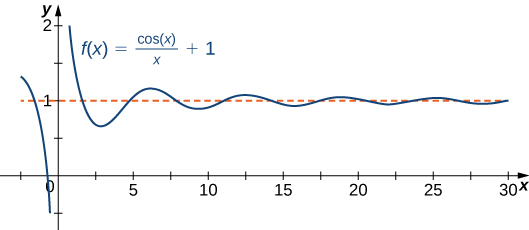Figure 3. The graph of $f(x)=\cos x/x+1$ crosses its horizontal asymptote $y=1$ an infinite number of times.

The algebraic limit laws and squeeze theorem we introduced in Introduction to Limits also apply to limits at infinity. We illustrate how to use these laws to compute several limits at infinity.

### Computing Limits at Infinity

For each of the following functions $f$, evaluate $\underset{x\to \infty }{\lim}f(x)$ and $\underset{x\to −\infty }{\lim}f(x)$. Determine the horizontal asymptote(s) for $f$.

1. $f(x)=5-\frac{2}{x^2}$
2. $f(x)=\frac{\sin x}{x}$
3. $f(x)= \tan^{-1} (x)$

Evaluate $\underset{x\to −\infty}{\lim}(3+\frac{4}{x})$ and $\underset{x\to \infty }{\lim}(3+\frac{4}{x})$. Determine the horizontal asymptotes of $f(x)=3+\frac{4}{x}$, if any.

#### Hint

$\underset{x\to \pm \infty }{\lim}1/x=0$

## Special Limits with Sine and Cosine

### Definition

$\underset{x\to \infty }{\lim}\sin x=DNE$                           $\underset{x\to \infty }{\lim}\cos x=DNE$                           $\underset{x\to \infty }{\lim}\frac{\sin x}{x}=\infty$                            $\underset{x\to \infty }{\lim}\frac{\cos x}{x}=\infty$

### Special limits with sine and cosine

For each of the following functions $f$, evaluate $\underset{x\to \infty }{\lim}f(x)$

1. $f(x)=\frac{2-\sin x}{x}$
2. $f(x)=\sin\left(\frac{1}{x}\right)$
3. $f(x)= \frac{6}{5x-\cos x}$

## Infinite Limits at Infinity

Sometimes the values of a function $f$ become arbitrarily large as $x\to \infty$ (or as $x\to −\infty )$. In this case, we write $\underset{x\to \infty }{\lim}f(x)=\infty$ (or $\underset{x\to −\infty }{\lim}f(x)=\infty )$. On the other hand, if the values of $f$ are negative but become arbitrarily large in magnitude as $x\to \infty$ (or as $x\to −\infty )$, we write $\underset{x\to \infty }{\lim}f(x)=−\infty$ (or $\underset{x\to −\infty }{\lim}f(x)=−\infty )$.

For example, consider the function $f(x)=x^3$. As seen in (Figure) and (Figure), as $x\to \infty$ the values $f(x)$ become arbitrarily large. Therefore, $\underset{x\to \infty }{\lim}x^3=\infty$. On the other hand, as $x\to −\infty$, the values of $f(x)=x^3$ are negative but become arbitrarily large in magnitude. Consequently, $\underset{x\to −\infty }{\lim}x^3=−\infty$.

 $x$ 10 20 50 100 1000 $x^3$ 1000 8000 125,000 1,000,000 1,000,000,000 $x$ -10 -20 -50 -100 -1000 $x^3$ -1000 -8000 -125,000 -1,000,000 -1,000,000,000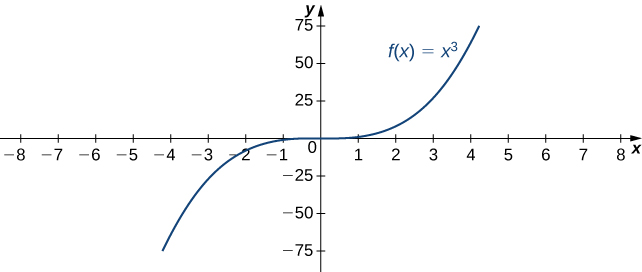Figure 8. For this function, the functional values approach infinity as $x\to \pm \infty$.

### Definition

(Informal) We say a function $f$ has an infinite limit at infinity and write

$\underset{x\to \infty }{\lim}f(x)=\infty$.

if $f(x)$ becomes arbitrarily large for $x$ sufficiently large. We say a function has a negative infinite limit at infinity and write

$\underset{x\to \infty }{\lim}f(x)=−\infty$.

if $f(x)<0$ and $|f(x)|$ becomes arbitrarily large for $x$ sufficiently large. Similarly, we can define infinite limits as $x\to −\infty$.

## Formal Definitions

Earlier, we used the terms arbitrarily close, arbitrarily large, and sufficiently large to define limits at infinity informally. Although these terms provide accurate descriptions of limits at infinity, they are not precise mathematically. Here are more formal definitions of limits at infinity. We then look at how to use these definitions to prove results involving limits at infinity.

### Definition

(Formal) We say a function $f$ has a limit at infinity, if there exists a real number $L$ such that for all $\epsilon >0$, there exists $N>0$ such that

$|f(x)-L|<\epsilon$

for all $x>N$. In that case, we write

$\underset{x\to \infty }{\lim}f(x)=L$

(see (Figure)).

We say a function $f$ has a limit at negative infinity if there exists a real number $L$ such that for all $\epsilon >0$, there exists $N<0$ such that

$|f(x)-L|<\epsilon$

for all $x<N$. In that case, we write

$\underset{x\to −\infty }{\lim}f(x)=L$.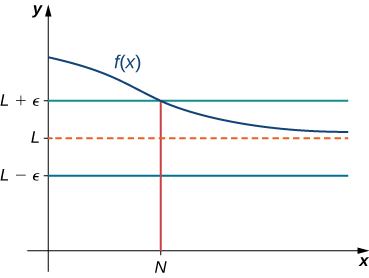Figure 9. For a function with a limit at infinity, for all $x>N$, $|f(x)-L|<\epsilon$.

Earlier in this section, we used graphical evidence in (Figure) and numerical evidence in (Figure) to conclude that $\underset{x\to \infty }{\lim}(\frac{2+1}{x})=2$. Here we use the formal definition of limit at infinity to prove this result rigorously.

### A Finite Limit at Infinity Example

Use the formal definition of limit at infinity to prove that $\underset{x\to \infty }{\lim}(2+\frac{1}{x})=2$.

Use the formal definition of limit at infinity to prove that $\underset{x\to \infty }{\lim}(3 - \frac{1}{x^2})=3$.

#### Hint

Let $N=\frac{1}{\sqrt{\epsilon }}$.

We now turn our attention to a more precise definition for an infinite limit at infinity.

### Definition

(Formal) We say a function $f$ has an infinite limit at infinity and write

$\underset{x\to \infty }{\lim}f(x)=\infty$

if for all $M>0$, there exists an $N>0$ such that

$f(x)>M$

for all $x>N$ (see (Figure)).

We say a function has a negative infinite limit at infinity and write

$\underset{x\to \infty }{\lim}f(x)=−\infty$

if for all $M<0$, there exists an $N>0$ such that

$f(x)<M$

for all $x>N$.

Similarly we can define limits as $x\to −\infty$.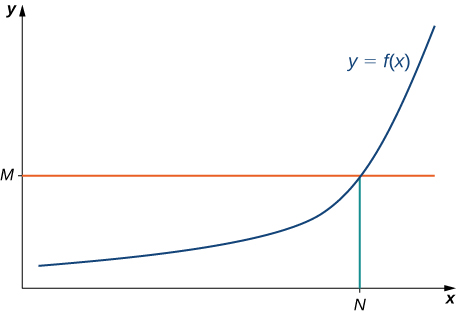Figure 10. For a function with an infinite limit at infinity, for all $x>N$, $f(x)>M$.

Earlier, we used graphical evidence ((Figure)) and numerical evidence ((Figure)) to conclude that $\underset{x\to \infty }{\lim}x^3=\infty$. Here we use the formal definition of infinite limit at infinity to prove that result.

### An Infinite Limit at Infinity

Use the formal definition of infinite limit at infinity to prove that $\underset{x\to \infty }{\lim}x^3=\infty$.

Use the formal definition of infinite limit at infinity to prove that $\underset{x\to \infty }{\lim}3x^2=\infty$.

#### Hint

Let $N=\sqrt{\frac{M}{3}}$.

# Key Equations

• Infinite Limits from the Left
$\underset{x\to a^-}{\lim}f(x)=+\infty$
$\underset{x\to a^-}{\lim}f(x)=−\infty$
• Infinite Limits from the Right
$\underset{x\to a^+}{\lim}f(x)=+\infty$
$\underset{x\to a^+}{\lim}f(x)=−\infty$
• Two-Sided Infinite Limits
$\underset{x\to a}{\lim}f(x)=+\infty: \underset{x\to a^-}{\lim}f(x)=+\infty$ and $\underset{x\to a^+}{\lim}f(x)=+\infty$
$\underset{x\to a}{\lim}f(x)=−\infty: \underset{x\to a^-}{\lim}f(x)=−\infty$ and $\underset{x\to a^+}{\lim}f(x)=−\infty$

In the following exercises, use direct substitution to obtain an undefined expression. Then, use the method of (Figure) to simplify the function to help determine the limit.

1. $\underset{x\to -2^-}{\lim}\frac{2x^2+7x-4}{x^2+x-2}$

2. $\underset{x\to -2^+}{\lim}\frac{2x^2+7x-4}{x^2+x-2}$

3. $\underset{x\to 1^-}{\lim}\frac{2x^2+7x-4}{x^2+x-2}$

4. $\underset{x\to 1^+}{\lim}\frac{2x^2+7x-4}{x^2+x-2}$

For the following exercises, evaluate the limit.

5. $\underset{x\to \infty }{\lim}\frac{1}{3x+6}$

6. $\underset{x\to \infty }{\lim}\frac{2x-5}{4x}$

7. $\underset{x\to \infty }{\lim}\frac{x^2-2x+5}{x+2}$

8. $\underset{x\to −\infty }{\lim}\frac{3x^3-2x}{x^2+2x+8}$

9. $\underset{x\to −\infty }{\lim}\frac{x^4-4x^3+1}{2-2x^2-7x^4}$

10. $\underset{x\to \infty }{\lim}\frac{3x}{\sqrt{x^2+1}}$

11. $\underset{x\to −\infty }{\lim}\frac{\sqrt{4x^2-1}}{x+2}$

12. $\underset{x\to \infty }{\lim}\frac{4x}{\sqrt{x^2-1}}$

13. $\underset{x\to −\infty }{\lim}\frac{4x}{\sqrt{x^2-1}}$

14. $\underset{x\to \infty }{\lim}\frac{2\sqrt{x}}{x-\sqrt{x}+1}$

For the following exercises, find the horizontal and vertical asymptotes.

15. $f(x)=x-\frac{9}{x}$

16. $f(x)=\frac{1}{1-x^2}$

17. $f(x)=\frac{x^3}{4-x^2}$

18. $f(x)=\frac{x^2+3}{x^2+1}$

19. $f(x)= \sin (x) \sin (2x)$

20. $f(x)= \cos x+ \cos (3x)+ \cos (5x)$

21. $f(x)=\frac{x \sin (x)}{x^2-1}$

$f(x)=\frac{x}{ \sin (x)}$

22. $f(x)=\frac{1}{x^3+x^2}$

23. $f(x)=\frac{1}{x-1}-2x$

24. $f(x)=\frac{x^3+1}{x^3-1}$

25. $f(x)=\frac{ \sin x+ \cos x}{ \sin x- \cos x}$

26. $f(x)=x- \sin x$

27. $f(x)=\frac{1}{x}-\sqrt{x}$

In the following exercises, sketch the graph of a function with the given properties.

28. $\underset{x\to -\infty }{\lim}f(x)=0, \, \underset{x\to -1^-}{\lim}f(x)=−\infty$, $\underset{x\to -1^+}{\lim}f(x)=\infty, \, \underset{x\to 0}{\lim}f(x)=f(0), \, f(0)=1, \, \underset{x\to \infty }{\lim}f(x)=−\infty$

29. $\underset{x\to -\infty}{\lim}f(x)=2, \, \underset{x\to 3^-}{\lim}f(x)=−\infty$, $\underset{x\to 3^+}{\lim}f(x)=\infty, \, \underset{x\to \infty }{\lim}f(x)=2, \, f(0)=\frac{-1}{3}$

30. $\underset{x\to -\infty }{\lim}f(x)=2, \, \underset{x\to -2}{\lim}f(x)=−\infty$,$\underset{x\to \infty }{\lim}f(x)=2, \, f(0)=0$

31. $\underset{x\to -\infty }{\lim}f(x)=0, \, \underset{x\to -1^-}{\lim}f(x)=\infty, \, \underset{x\to -1^+}{\lim}f(x)=−\infty, \, f(0)=-1, \, \underset{x\to 1^-}{\lim}f(x)=−\infty, \, \underset{x\to 1^+}{\lim}f(x)=\infty, \, \underset{x\to \infty }{\lim}f(x)=0$

32. [T] In physics, the magnitude of an electric field generated by a point charge at a distance $r$ in vacuum is governed by Coulomb’s law: $E(r)=\large \frac{q}{4\pi \epsilon_0 r^2}$, where $E$ represents the magnitude of the electric field, $q$ is the charge of the particle, $r$ is the distance between the particle and where the strength of the field is measured, and $\large \frac{1}{4\pi \epsilon_0}$ is Coulomb’s constant: $8.988 \times 10^9 \, \text{N} \cdot \text{m}^2/\text{C}^2$.

1. Use a graphing calculator to graph $E(r)$ given that the charge of the particle is $q=10^{-10}$.
2. Evaluate $\underset{r\to 0^+}{\lim}E(r)$. What is the physical meaning of this quantity? Is it physically relevant? Why are you evaluating from the right?

33. [T] The density of an object is given by its mass divided by its volume: $\rho =m/V$.

1. Use a calculator to plot the volume as a function of density $(V=m/\rho)$, assuming you are examining something of mass 8 kg ($m=8$).
2. Evaluate $\underset{\rho \to 0^+}{\lim}V(\rho)$ and explain the physical meaning.